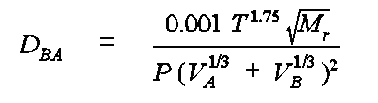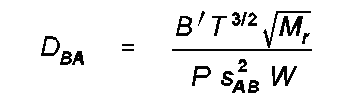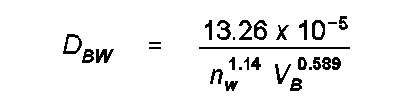# EPA On-line Tools for Site Assessment Calculation

The estimates of diffusion coefficients are developed from methods presented in Chapter 17: Diffusion Coefficients in Air and Water by William A. Tucker and Leslie H. Nelken of the "Handbook of Chemical Property Estimation Methods" by Warren J. Lyman, William F. Reehl and David H. Rosenblatt, first edition, 1990, American Chemical Society.

The calculator uses three methods to make estimates of diffusion coefficients in air (FSG, FSG-LaBas and WL) and one method for the diffusion coefficient in water (HL). Each of these are described below, with complete details given the reference.

### Diffusion Coefficients in Air

FSG The Fuller, Schettler and Giddings (FSG) method is based on the regression formula:where DBA is the diffusion coefficient of compound B in compound A in cm2/s, T is the temperature in K, P is the pressure in atm, Mr a function of the molecular weights MA and MB of compounds A and B, VA and VB are the molar volumes of air (A) and the gas (B) in question. Mr is equal to (MA + MB)/MA MB. VB can be estimated from volume increments associated with each element in the compound. These increments give the volume (cm3) per mole of atom present. The values are given in the reference and have been programmed into the calculator.

FSG-LaBas The FSG-LaBas method uses the same formula as the FSG method but substitutes the LaBas volume estimates for molar volume. The FSG-LaBas method allows for estimating the diffusivities of more compounds of interest.

WL The Wilke and Lee (WL) method uses LaBas molar volumes and is based upon a series of calculations of a "collision integral" W that represents collision between atoms. The estimate of DBA is given bywhere B' is a function of the molecular weights of A and B, s BA 2 is the average molal volume at the boiling point of A and B, W is the collision integral. The details of calculation of these quantities are given in the reference.

### Diffusion Coefficients in Water

HL The Hayduk and Laudie (HL) method for estimating the diffusivity of an organic compound in water in cm2/s is given bywhere DBA is the diffusion coefficient of compound B in compound A (water) in cm2/s, nw is the viscosity of water in cp (corrected for temperature) and V_B are the LaBas molar volume increments.

 Previous Top ^ Next

Home | Glossary | Notation | Links | References | Calculators

Contact Jim Weaver to ask a technical question on this material.